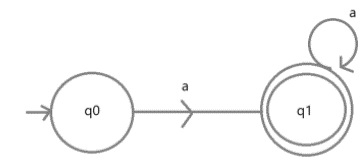# C Program to construct a DFA which accepts L = {aN | N â‰¥ 1}

Let us take a string S of size N, we have to design a Deterministic Finite Automata (DFA) for accepting the language L = {aN | N ≥ 1}.

The string accepting the language L is {a, aa, aaa, aaaaaaa…, }.

Now the user has to enter a string, if that string is present in the given language, then print “entered string is Accepted”. Otherwise, print “entered string is not Accepted”.

DFA transition diagram for the given language is## Example

Following is the C program to construct DFA which accepts the language L = {aN | N ≥ 1}

#include<stdio.h>
int main() {
char S;
int l,i;
int count = 0;
printf("To implement DFA of language {aN | N ≥ 1}  enter input string:");
scanf("%s",S);
l=strlen(S);
for (i=0;i<l;i++) {
if(S[i]!='a') {
printf("entered string is NOT ACCEPTED");
getch();
exit(0);
}
if (S[i] == 'a')
count++;
else
printf("invalid input");
}
if (count == l && count != 0) {
printf("entered string is accepted");
}
return 0;
}

## Output

You will get the following output −

Run 1:
To implement DFA of language {aN | N >= 1}
enter input string:aaaa
entered string is accepted
Run 2:
To implement DFA of language {aN | N >= 1}
entered string is NOT ACCEPTED